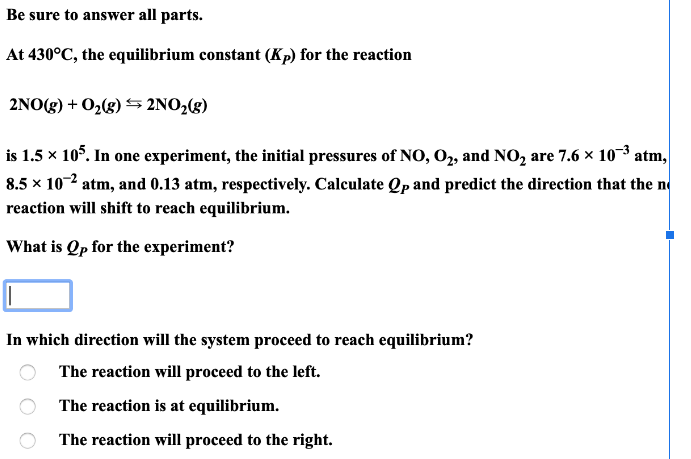# At 430 degrees C, the equilibrium constant (Kp) for the reaction 2NO(g) + O2(g) ⇌ 2NO2(g) is 1.5x10^5. In one experiment, the initial pressures of NO, O2, and NO2 are 7.6x10^-3 atm, 8.5x10^-2 atm, and 0.13 atm, respectively. Calculate Qp and predict the direction that the reaction will shift to reach equilibrium. What is Qp for the experiment? In which direction will the system proceed to reach equilibrium?